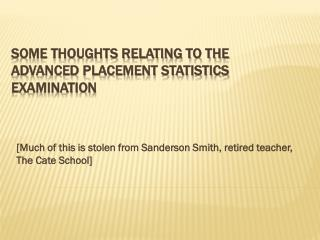DownloadDownload PresentationSOME THOUGHTS RELATING TO THE ADVANCED PLACEMENT STATISTICS EXAMINATION

# SOME THOUGHTS RELATING TO THE ADVANCED PLACEMENT STATISTICS EXAMINATION

Download Presentation## SOME THOUGHTS RELATING TO THE ADVANCED PLACEMENT STATISTICS EXAMINATION

- - - - - - - - - - - - - - - - - - - - - - - - - - - E N D - - - - - - - - - - - - - - - - - - - - - - - - - - -
##### Presentation Transcript

1. [Much of this is stolen from Sanderson Smith, retired teacher, The Cate School] SOME THOUGHTS RELATING TO THEADVANCED PLACEMENT STATISTICS EXAMINATION

2. Graphing calculators are required (not just allowed) for this exam. You may use it on any problem throughout the testing period, but many of the problems are not dependent on computations. • For the first 90 minutes, you will work on 40 multiple choice questions. • After a break, you will work on 6 free response questions for another 90 minutes. The first five problems account for 75% of your free response score, and Problem 6 by itself is 25% of your free response score. The Structure of the Exam

3. Relax, and think. Remember that everyone else taking the exam is in a situation identical to yours. Realize that the problems will probably look detailed and verbose compared to problems you have encountered in other math courses. Make sure your calculator is functioning properly. Insert new batteries a day or so before the exam, and make sure all systems are "go." Bring a backup calculator, if possible. Miscellaneous advice

4. Problem 1 is a graphical display problem (which we’ve practiced). Do this first. Problem 6 is the Investigative Task. Try that second and spend about 20-25 minutes on it. When you’ve done all you can on Problem 6 (or get stuck), go back through Problems 2 – 5. Clear and concise communication is always important. Attacking the 6 free response problems

5. Don't confuse median and mean. They are both measures of center, but, for a given data set, they may differ by a considerable amount. mean > median distribution skewed right mean < median distribution skewed left Mean and median

6. A slope close to 1 or -1 doesn't mean strong correlation. An r value close to 1 or -1 doesn't mean the slope of least-squares regression line is close to 1 or -1. Relation between b1 (slope of regression line) and r (coefficient of correlation) is Remember that r2 > 0 doesn't mean r > 0. For instance, if r2 = 0.81, then r = 0.9 or r = -0.9. Correlation coefficient and The least-squares regression line.

7. Correlation coefficient and The least-squares regression line. • Remember that the least-squares regression line contains the point , i.e. the mean of the x-values and the mean of the y-values. • A coefficient of correlation near 0 doesn't necessarily mean there are no meaningful relationships to be observed between the two data sets. In this case, r = .38, but a scatterplot displays something quite interesting. Moral of story: Whenever possible, look at the "shape" of the data.

8. Even if each individual in a group has an equal probability of being chosen in a sample, it doesn't follow that the sample is an SRS. • Consider a class of 6 boys and 6 girls. I want to randomly pick one boy and one girl from this group using a die. Note that each child has the same probability (1/6) of being chosen. • However, the chosen two students do not represent an SRS of size two picked from members of the class. Can you explain why? Be careful with the concept of simple random sample (SRS).

9. Be aware of numerical statistical changes when transformations are made on a data set, W. Adding the same number to each number in W simply shifts the data. This doesn't change standard deviation and variance. Multiplying all numbers in W by a constant does change standard deviation and variance Watch!.... Transformations of random variables

10. Simple examples… start with set sx Add 7 to each data value: Multiply each data value by 4: Multiply by 4 and add 7 to each data value:

11. (a) When combining two independent sets by addition, means add; standard deviations do not add; variances add. (b) When combining two independent sets by subtraction, means subtract; standard deviations do not subtract; variances still add. Random variables

12. Thinking in terms of slots, if you have a set number of slots, and the probability of getting a "success" in each slot is constant, then you have a binomial setting. Consider, for instance, rolling a die ten times. There are ten slots to be filled, and the probability of filling any slot with the outcome "6" is a constant 1/6. Recognize a binomial distribution

13. The probability of getting exactly three 6's in ten rolls is binompdf(10,1/6,3) = 0.155045, or about 15.5%. The probability of getting less than four 6's is binomcdf(10,1/6,3) = 0.93027, or about 93%. The probability of getting four or more 6's in 10 rolls of a single die is then about 1 – 0.93 = 7% Binomial computations

14. The cumulative frequency for a value x is the percent of scores that are less than or equal to x. This a graph of the heights of college basketball players. Reading a cumulative Relative frequency graph

15. The 25th percentile (or first quartile) is about 71 inches The 75th percentile (or third quartile) is about 77 inches. The IQR is then 6 inches. What is the median? Reading a cumulative Relative frequency graph